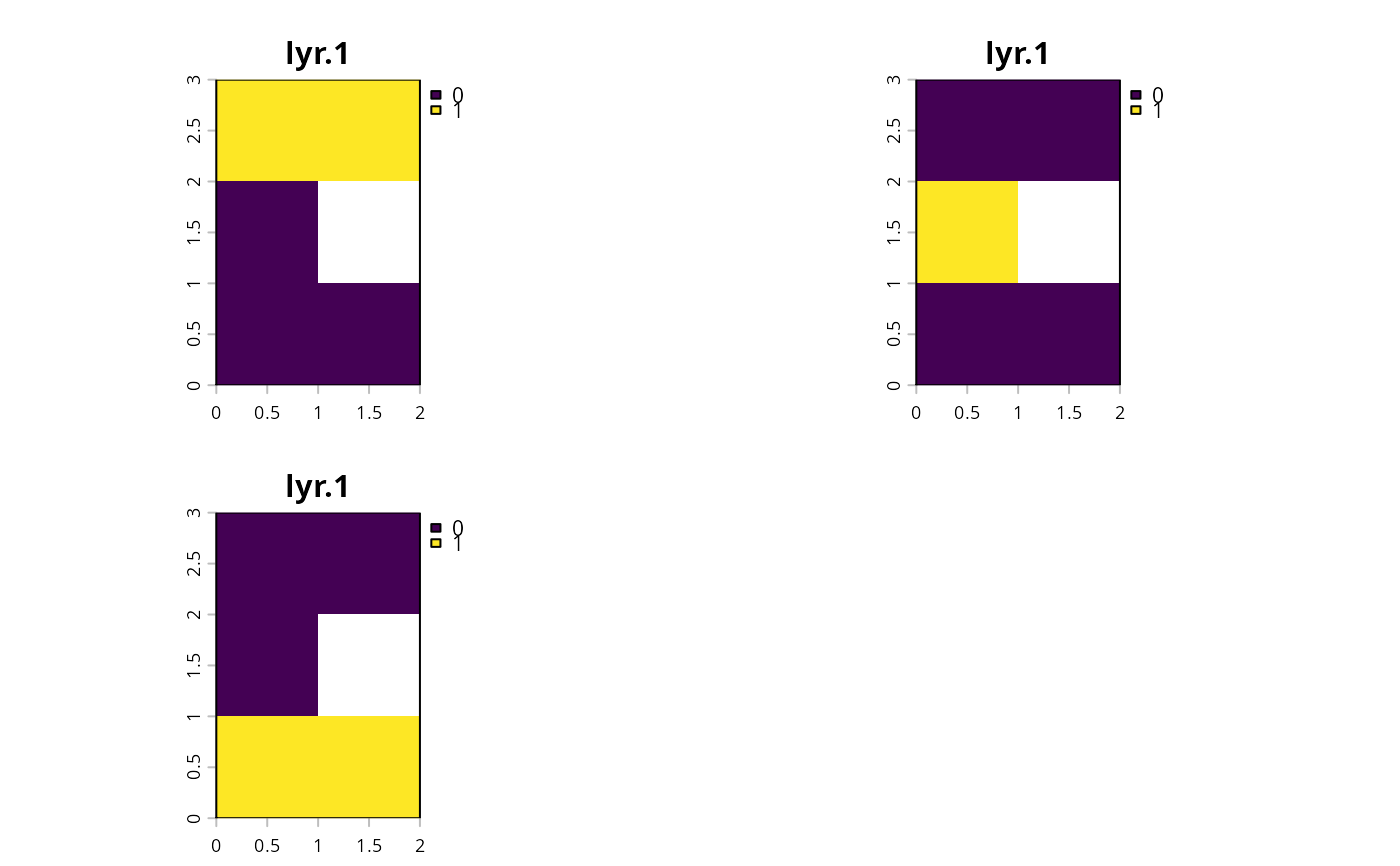Convert a RasterStack object where each layer corresponds to a different identifier and values indicate the presence/absence of that category into a RasterLayer object containing categorical identifiers.

category_layer(x)

## Arguments

x

Raster object containing a multiple layers. Note that pixels must be 0, 1 or NA values.

## Value

RasterLayer object.

## Details

This function is provided to help manage data that encompass multiple management zones. For instance, this function may be helpful for interpreting solutions for problems associated with multiple zones that have binary decisions.

binary_stack().

## Examples

# create a binary raster stack
x <- stack(raster(matrix(c(1, 0, 0, 1, NA, 0), nrow = 3)),
raster(matrix(c(0, 1, 0, 0, NA, 0), nrow = 3)),
raster(matrix(c(0, 0, 1, 0, NA, 1), nrow = 3)))

# convert to binary stack
y <- category_layer(x)

# plot categorical raster and binary stack representation
# \dontrun{
plot(stack(x, y), main = c("x[]", "x[]", "x[]", "y"),
nr = 1)# }